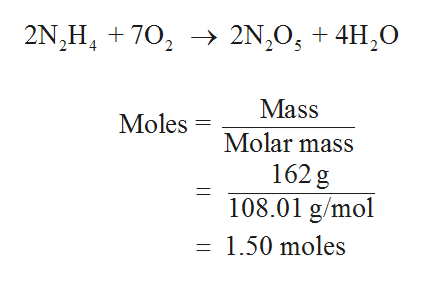# In the following reaction, how many grams of O2 must be reacted in order to produce 162 grams of N2O5 (MW = 108.0 g/mol) at a 80.0% yield? 2 N2H4(g) + 7 O2(g) → 2 N2O5(g) + 4 H2O(g)

Question
28 views

In the following reaction, how many grams of O2 must be reacted in order to produce 162 grams of N2O5 (MW = 108.0 g/mol) at a 80.0% yield? 2 N2H4(g) + 7 O2(g) → 2 N2O5(g) + 4 H2O(g)

check_circle

Step 1

First, the given N2O5 grams is converted into moles. The given ...help_outlineImage Transcriptionclose2N,0 4HO 2N H70 Mass Moles Molar mass 162 g 108.01 g/mol = 1.50 moles fullscreen

### Want to see the full answer?

See Solution

#### Want to see this answer and more?

Solutions are written by subject experts who are available 24/7. Questions are typically answered within 1 hour.*

See Solution
*Response times may vary by subject and question.
Tagged in

### Chemistry